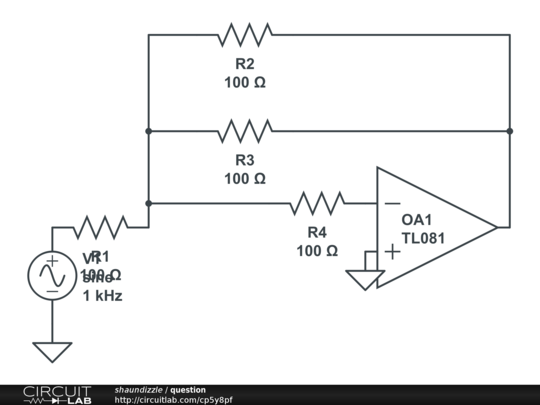# Findout output voltage of an opamp when given input voltage and open loop gain

Just in theory, please ignore the values...

Given the open loop gain of an opamp and its input voltage and a circuit similar to the figure below, how would I find the output voltage?]

My thoughts were to just do nodal analysis and avoid the open loop gain all together since the circuit given is closed loop, I thought that it was irrelevant and just given to throw you off. Or would the correct steps be to assume: v_out = A(v_+ - v_-) v_out = A(-v_-)

• Use ideal OpAmp assumptions. Oct 20 '15 at 1:49
• @NickAlexeev So then we would assume v+ = v-, and A = inf. And we would just find v_out using nodal analysis... right? Oct 20 '15 at 2:18
• There is also the second ideal opamp assumption: The inputs of the ideal OpAmp draw no current. You are on the right track, though. Oct 20 '15 at 2:31
• @NickAlexeev Nice. Ok, yeah I am familiar with the assumptions for an ideal opamp, I was just curious about the assumption of what to do with the open loop gain. Like I said, I thought we should ignore that since our circuit is close loop (i.e. we have feedback). Thanks Oct 20 '15 at 2:51
• The schematic doesn't show any op amps. Did you make changes to it in CircuitLab after posting the question? Oct 20 '15 at 3:41

As already noted, your circuit simplifies to:When you close the loop the open-loop gain is reduced to the ratio $\frac{\text R2}{\text R1}$ so, since it's an inverting amplifier, the output voltage becomes: $\ \ \text {Vout} = \ \ - \frac {\text R2}{\text R1} \times \text{Vin}$